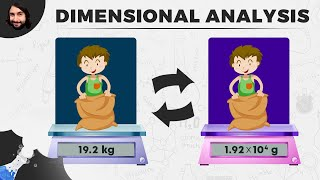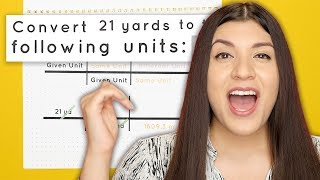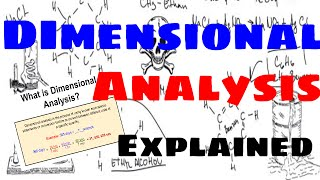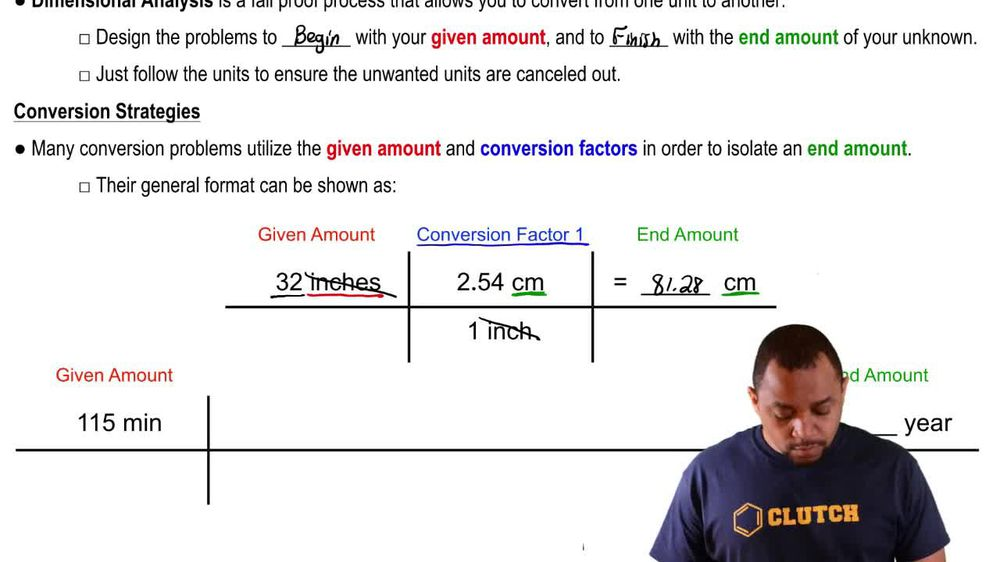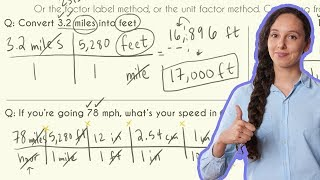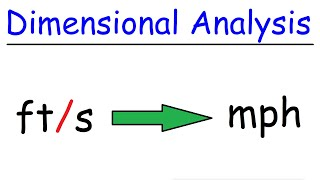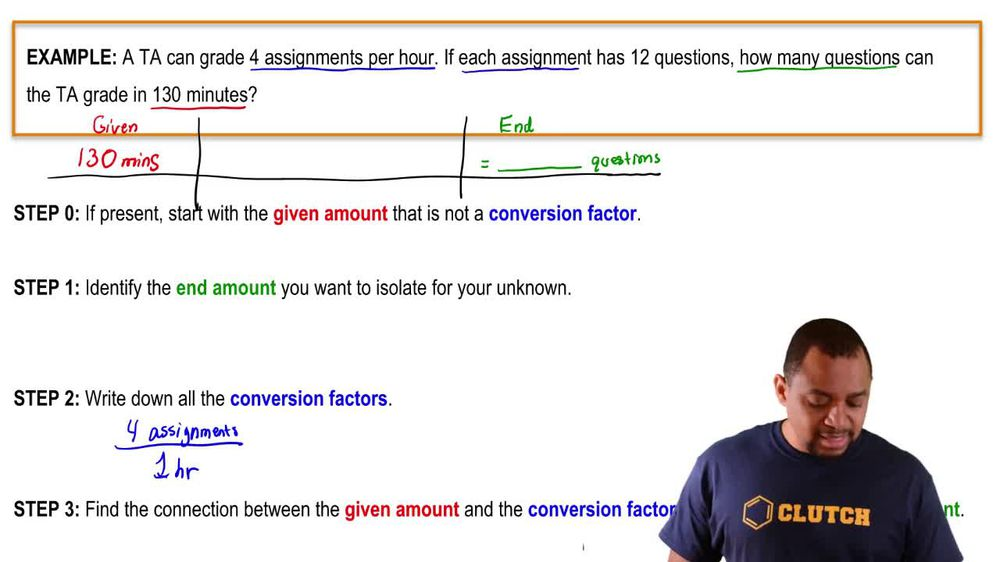Start typing, then use the up and down arrows to select an option from the list.
1. 1. Intro to General Chemistry2. Dimensional Analysis
Problem

# Complete the table. a. 355 km>s _____cm>s _____m>ms b. 1228 g>l _____g>ml _____kg>ml c. 554 mK>s _____K>s _____mK>ms d. 2.554 mg>ml _____g>l _____mg>ml

Relevant Solution3m
Play a video:
Hello, everyone in this video, we're trying to convert the units of gallons permanent into milliliters per hour. So first I know that we're given the 3. gallons per minute and the value that they do not write in is going to be one because we know that any value divide by one is going to be itself. So typically we don't write that one, but I want to go ahead, fill that in just so the dimensional analysis looks less empty and maybe make more sense. So we're trying to go from the gallons per minute into milliliters per hour. So we can see that we're trying to convert not only the gallon units, but we're also trying to convert the Denominator unit. So we have time and volume to consider. We don't have to do everything at once. We do it one step at a time. Maybe to convert the guns two ml first or minutes to our doesn't really matter because multiplication is cumulative. So everything will eventually just kind of cancel each other out, divide out whatever it is and we should be able to find the male layers per hour. So just one at a time. So Starting off my dimension analysis, I'm going to be using the only value that they gave me, which is my 3.03 gallons per one minutes. So I have decided that I'm going to first convert my volume unit, which is my gallons into the desired milliliters. I don't know any direct um conversion into milliliters. So I do baby steps. So first I'm gonna go ahead and convert gallons into leaders. So I know that everyone gallon, There's going to be 3. l And then I can go from leaders into ml. So I know that everyone male leader is going to equal to 10 to the negative three leaders. So we can see now that my gallons will cancel and leaders will cancel leaving me with the male leaders on top, which is exactly what we want. So now that we have tackled down that volume unit, we can now focus on our time unit which is to convert from minutes into hours. So I can go ahead and do a direct conversion for this one because I know that conversion, I know that from every one hour we have minutes. Right? So you can see here the minute will cancel out and we're left with now the hours on the bottom exactly what we want. So plugging in all these numerical values by dividing multiplying and all that, we can get a value of 6. Times 10 to the 5th power and units will be familiar on top and hours on bottom. So milliliters per hour, right? Milly's on top, hours on bottom. And that's going to be my final answer for this problem